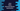# Java Math decrementExact explanation with example## Java Math decrementExact :

Java decrementExact is a utility method defined in java.lang.Math class. We can pass one argument and it returns the argument decremented by one. This method is available for integer and long argument types.

In this post, I will show you how to use this method and its corner cases with examples.

### Definitions :

decrementExact is defined as below :

``static int decrementExact(int arg)``

and

``static int decrementExact(long arg)``

Both are static methods. So, we can use them without creating a new object of the Math class. These methods will throw one exception if the result overflows an integer or long value.

### Example of decrementExact :

Let’s consider the below example :

``````public class Main {
public static void main(String[] args) {
System.out.println(Math.decrementExact(100));
System.out.println(Math.decrementExact(Integer.MAX_VALUE));

System.out.println(Math.decrementExact(1000L));
System.out.println(Math.decrementExact(Long.MAX_VALUE));
}
}``````

It will print the below output :

``````99
2147483646
999
9223372036854775806``````

Here, we are using the decrementExact method for both integer and long arguments. We are also using it with the max value of integer and long.

### Example of decrementExact with Exception :

decrementExact throws one exception if the result overflows the value of integer or long. For example :

``````public class Main {
public static void main(String[] args) {
//System.out.println(Math.decrementExact(Integer.MIN_VALUE));

System.out.println(Math.decrementExact(Long.MIN_VALUE));
}
}``````

It will throw one exception, ArithmeticException. Because we can’t decrement below the minimum value of integer or long. If you execute the above program, it will throw long overflow ArithmeticException and if you remove the comment, it will throw int overflow ArithmeticException.